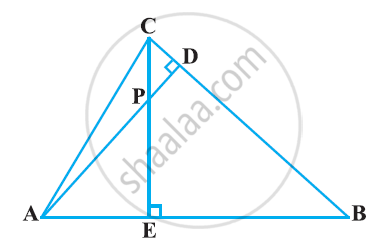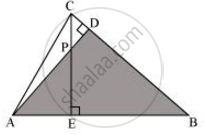Share

# In the following figure, altitudes AD and CE of ΔABC intersect each other at the point P. Show that - CBSE Class 10 - Mathematics

ConceptCriteria for Similarity of Triangles

#### Question

In the following figure, altitudes AD and CE of ΔABC intersect each other at the point P. Show that:#### Solution∠PAE = ∠DAB (Common)

Hence, by using AA similarity criterion,